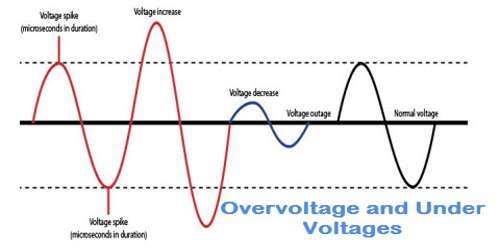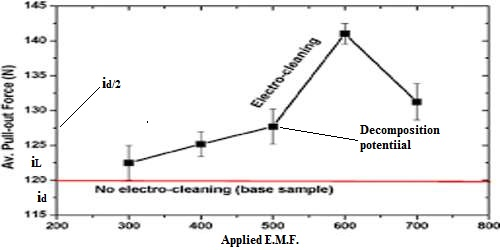# Overvoltage

Overvoltage

The cell reaction can be reversed when we apply the opposing emf. Usually the opposing voltage required to reverse the cell reaction is much greater than the emf of the cell. For example, consider a cell,

Pt / H2 (1 atm) / HCl (a=1) / Pt / O2 (1-atm)

The minimum e.m.f at which the current flows freely corresponds to that at which bubbles of gas are first observed on the electrode. This minimum e.m.f. that must be applied to bring about continuous electrolysis of the solution of a particular substance is known as the decomposition voltage (also known as discharge potential) of the electrolyte.

The voltage of this cell is 1.12 volt. If the opposing voltage is slightly greater than 1.12 the cell reaction should get reversed and evolution of H2 and O2 should start. But in actual practice, it is not true, an opposing emf of 1.7 volts is required or in excess of 1.7 – 1.12 = 0.58 volt is required to cause the reverse reaction.

This excess of voltage, over the voltage of the cell that is necessary to cause the reverse reaction is called as over voltage.As can be seen from Figure.

(i) the (i vs E) curve starts from zero and with increasing potential a small current flows till the decomposition potential as reached.

(ii) the rate of discharge becomes appreciable with slight increase of e.m.f., and the current and the rate of diffusion also increase significantly.

(iii) there is a limit to the rate of diffusion (which also depends on concentration), and as soon as this point is reached further increase of potential does not cause an further increase of current.

The current corresponding to the limiting rate of diffusion is called the limiting current (iL). The small current that flows through the cell before the decomposition potential is reached is called residual current (ir). The difference between the limiting current and residual current is called the diffusion current (id). The potential corresponding to the half of the diffusion current is called half-wave potential (id/2). The half-wave potential is characteristic of a particular ion, and the polarographic method of analysis is based on this principle.

It is found that for most strong acids and bases the decomposition voltage is about 1.7 volts. In a reversible cell containing acids and bases as electrolytes the evolution of gas would theoretically start when the voltage is 1.2. The difference between this potential and the measured decomposition potential is known as the over-voltage. The overvoltage depends, besides other factors, on current density and on the nature of the electrode. It may be observed that overvoltage is due to polarization of the electrode.

The overvoltage is important in practical applications of electrochemistry. The high voltage of the lead storage cell is due to the user voltage of lead. Electro-deposition from acid solution of certain metals, such as zinc, tin or cadmium is possible in spite of their relatively high position in the electromotive series.